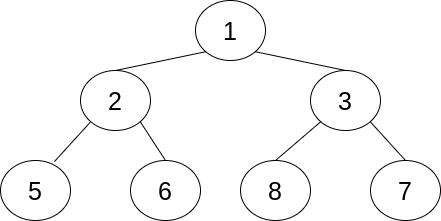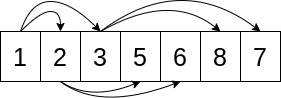# The Python heapq Module: Using Heaps and Priority Queues

by Moshe Zadka Jun 24, 2020 intermediate python

Heaps and priority queues are little-known but surprisingly useful data structures. For many problems that involve finding the best element in a dataset, they offer a solution that’s easy to use and highly effective. The Python `heapq` module is part of the standard library. It implements all the low-level heap operations as well as some high-level common uses for heaps.

A priority queue is a powerful tool that can solve problems as varied as writing an email scheduler, finding the shortest path on a map, or merging log files. Programming is full of optimization problems in which the goal is to find the best element. Priority queues and the functions in the Python `heapq` module can often help with that.

In this tutorial, you’ll learn:

• What heaps and priority queues are and how they relate to each other
• What kinds of problems can be solved using a heap
• How to use the Python `heapq` module to solve those problems

This tutorial is for Pythonistas who are comfortable with lists, dicts, sets, and generators and are looking for more sophisticated data structures.

## What Are Heaps?

Heaps are concrete data structures, whereas priority queues are abstract data structures. An abstract data structure determines the interface, while a concrete data structure defines the implementation.

Heaps are commonly used to implement priority queues. They’re the most popular concrete data structure for implementing the priority queue abstract data structure.

Concrete data structures also specify performance guarantees. Performance guarantees define the relationship between the size of the structure and the time operations take. Understanding those guarantees allows you to predict how much time the program will take as the size of its inputs change.

### Data Structures, Heaps, and Priority Queues

Abstract data structures specify operations and the relationships between them. The priority queue abstract data structure, for example, supports three operations:

1. is_empty checks whether the queue is empty.
3. pop_element pops the element with the highest priority.

Priority queues are commonly used for optimizing task execution, in which the goal is to work on the task with the highest priority. After a task is completed, its priority is lowered, and it’s returned to the queue.

There are two different conventions for determining the priority of an element:

1. The largest element has the highest priority.
2. The smallest element has the highest priority.

These two conventions are equivalent because you can always reverse the effective order. For example, if your elements consist of numbers, then using negative numbers will flip the conventions around.

The Python `heapq` module uses the second convention, which is generally the more common of the two. Under this convention, the smallest element has the highest priority. This might sound surprising, but it’s often quite useful. In the real-life examples you’ll see later, this convention will simplify your code.

Concrete data structures implement the operations defined in an abstract data structure and further specify performance guarantees.

The heap implementation of the priority queue guarantees that both pushing (adding) and popping (removing) elements are logarithmic time operations. This means that the time it takes to do push and pop is proportional to the base-2 logarithm of the number of elements.

Logarithms grow slowly. The base-2 logarithm of fifteen is about four, while the base-2 logarithm of a trillion is about forty. This means that if an algorithm is fast enough on fifteen elements, then it’s going to be only ten times slower on a trillion elements and will probably still be fast enough.

In any discussion of performance, the biggest caveat is that these abstract considerations are less meaningful than actually measuring a concrete program and learning where the bottlenecks are. General performance guarantees are still important for making useful predictions about program behavior, but those predictions should be confirmed.

### Implementation of Heaps

A heap implements a priority queue as a complete binary tree. In a binary tree, each node will have at most two children. In a complete binary tree, all levels except possibly the deepest one are full at all times. If the deepest level is incomplete, then it will have the nodes as far to the left as possible.

The completeness property means that the depth of the tree is the base-2 logarithm of the number of elements, rounded up. Here’s an example of a complete binary tree:In this particular example, all levels are complete. Each node except for the deepest ones has exactly two children. There are a total of seven nodes in three levels. Three is the base-2 logarithm of seven, rounded up.

The single node at the base level is called the root node. It might seem weird to call the node at the top of the tree the root, but this is the common convention in programming and computer science.

The performance guarantees in a heap depend on how elements percolate up and down the tree. The practical result of this is that the number of comparisons in a heap is the base-2 logarithm of the size of the tree.

In a heap tree, the value in a node is always smaller than both of its children. This is called the heap property. This is different from a binary search tree, in which only the left node will be smaller than the value of its parent.

The algorithms for both pushing and popping rely on temporarily violating the heap property, then fixing the heap property through comparisons and replacements up or down a single branch.

For example, to push an element onto a heap, Python adds the new node to the next open slot. If the bottom layer isn’t full, then the node is added to the next open slot at the bottom. Otherwise, a new level is created and then the element is added to the new bottom layer.

Once the node is added, Python compares it to its parent. If the heap property is violated, then the node and its parent are switched, and the check begins again at the parent. This continues until the heap property holds or the root has been reached.

Similarly, when popping the smallest element, Python knows that, because of the heap property, the element is at the root of the tree. It replaces the element with the last element at the deepest layer and then checks if the heap property is violated down the branch.

### Uses of Priority Queues

A priority queue, and a heap as an implementation of a priority queue, is useful for programs that involve finding an element that is extreme in some way. For example, you can use a priority queue for any of the following tasks:

• Getting the three most popular blog posts from hit data
• Finding the fastest way to get from one point to the other
• Predicting which bus will be the first to arrive at a station based on arrival frequency

Another task for which you could use a priority queue is scheduling emails. Imagine a system that has several kinds of emails, each of which needs to be sent at a certain frequency. One kind of email needs to go out every fifteen minutes, and another needs to be sent every forty minutes.

A scheduler could add both types of email to the queue with a timestamp indicating when the email next needs to be sent. Then the scheduler could look at the element with the smallest timestamp—indicating that it’s next in line to be sent—and calculate how long to sleep before sending.

When the scheduler wakes up, it would process the relevant email, take the email out of the priority queue, calculate the next timestamp, and put the email back in the queue at the correct location.

## Heaps as Lists in the Python `heapq` Module

Although you saw the heap described earlier as a tree, it’s important to remember that it’s a complete binary tree. Completeness means that it’s always possible to tell how many elements are at each layer except the last one. Because of this, heaps can be implemented as a list. This is what the Python `heapq` module does.

There are three rules that determine the relationship between the element at the index `k` and its surrounding elements:

1. Its first child is at `2*k + 1`.
2. Its second child is at `2*k + 2`.
3. Its parent is at `(k + 1) // 2`.

The rules above tell you how to visualize a list as a complete binary tree. Keep in mind that an element always has a parent, but some elements don’t have children. If `2*k` is beyond the end of the list, then the element doesn’t have any children. If `2*k` is a valid index but `2*k + 1` is not, then the element has only one child.

The heap property means that if `h` is a heap, then the following will never be `False`:

``````h[k] <= h[2*k + 1] and h[k] <= h[2*k + 2]
``````

It might raise an `IndexError` if any of the indices is beyond the length of the list, but it will never be `False`.

In other words, an element must always be smaller than the elements that are at twice its index plus one and twice its index plus two.

Here’s a visual of a list that satisfies the heap property:The arrows go from element `k` to elements `2*k + 1` and `2*k + 2`. For example, the first element in a Python list has the index `0`, so its two arrows point at indices `1` and `2`. Notice how the arrows always go from a smaller value to a bigger value. This is how you can check that the list satisfies the heap property.

### Basic Operations

The Python `heapq` module implements heap operations on lists. Unlike many other modules, it does not define a custom class. The Python `heapq` module has functions that work on lists directly.

Usually, as in the email example above, elements will be inserted into a heap one by one, starting with an empty heap. However, if there’s already a list of elements that needs to be a heap, then the Python `heapq` module includes `heapify()` for turning a list into a valid heap.

The following code uses `heapify()` to turn `a` into a heap.

::...#### 自怼圈/年度番新